﻿ The Use of Digital Image Correlation Method in Contact Mechanics

### The Use of Digital Image Correlation Method in Contact Mechanics

Martin Hagara, Pavol Lengvarský, Jozef Bocko, Peter Pavelka

American Journal of Mechanical Engineering

## The Use of Digital Image Correlation Method in Contact Mechanics

Martin Hagara1,, Pavol Lengvarský1, Jozef Bocko1, Peter Pavelka1

1Department of Applied Mechanics and Mechanical Engineering, Faculty of Mechanical Engineering, Technical University of Košice, Košice, Slovakia

### Abstract

This paper deals with the description of methodology for experimental non-contact investigation of stress fields in the area of contact mechanics using digital image correlation method. The measurement was realized in laboratory conditions via low-speed digital image correlation system. As the control software of mentioned digital image correlation system does not allow investigate and visualize stress fields, the authors used an additional application called Q-STRESS v.1.0 allowing compute several types of stress. The paper also briefly described the creation of numerical model in Ansys software. The Von Mises stress levels were in chosen location compared, whereby the results show agreement adequate for comparison of experimental and numerical modeling.

• Martin Hagara, Pavol Lengvarský, Jozef Bocko, Peter Pavelka. The Use of Digital Image Correlation Method in Contact Mechanics. American Journal of Mechanical Engineering. Vol. 4, No. 7, 2016, pp 445-449. http://pubs.sciepub.com/ajme/4/7/39
• Hagara, Martin, et al. "The Use of Digital Image Correlation Method in Contact Mechanics." American Journal of Mechanical Engineering 4.7 (2016): 445-449.
• Hagara, M. , Lengvarský, P. , Bocko, J. , & Pavelka, P. (2016). The Use of Digital Image Correlation Method in Contact Mechanics. American Journal of Mechanical Engineering, 4(7), 445-449.
• Hagara, Martin, Pavol Lengvarský, Jozef Bocko, and Peter Pavelka. "The Use of Digital Image Correlation Method in Contact Mechanics." American Journal of Mechanical Engineering 4, no. 7 (2016): 445-449.

 Import into BibTeX Import into EndNote Import into RefMan Import into RefWorks

123
Prev Next

### 1. Introduction to Contact Mechanics

Contact mechanics belongs to a group of basic disciplines in mechanical engineering. There are a lot of cases, in which contact between two bodies appears, e.g. gear wheels, rolling bearings, brakes or trains and rails. Contact mechanics deals with deformation analysis of bodies, which act to each other. During some last decades a lot of analyses were performed in order to find a right model for contact between bodies, as real as possible. Although the knowledge of contact and friction principles has been used since the early history, the first, who started to concern with contact mechanics, was Heinrich Hertz in 1882. Hertz performed the first analytical study of contact between two smoothed spherical bodies, which deform elastically. He did not consider any friction or adhesive forces. These simplifications cause, that this model can be in some cases inaccurate or inapplicable. His theory was improved by Johnson, Kendall and Roberts . Their model, known as JKR model, considered also surface forces acting between the bodies. The other alternative of Hertz model was DMT model introduced by Derjaguin, Muller and Toporov . Both approaches were subsequently improved by Maugis, which model can be applicable for various materials with low or high adhesion. Modern discipline, which concerns with the body contact, their friction and abrasions, is known as tribology [3, 4, 5].

### 2. Hertzian Contact Theory

Hertz considered such assumptions :

• the contact surface size compared with the body size is too small,

• both contact faces are smoothed and without friction,

• the stresses caused by material deformation are elastic,

• the gap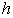outside the location of surface deformation can be expressed as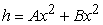, where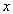and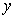are the coordinates lying on the mutual tangent plane to both surfaces.

The Hertzian contact theory is valid for contact of two spheres, cylinders or ellipsoids.

The roughness of surfaces is also an important aspect, which affects the values of contact stress. Therefore Hertzian model considers a simplification for roughness, which assumes that the surface of bodies in contact is created by regular round ruggednesses with the same radiuses of curvature and heights, as can be seen in Figure 1.Download asVeiw figureFigures index
Figure 1. Roughness considered by Hertzian contact theory
2.1. Contact of Round-Shaped Bodies

Let us consider two elastic spherical bodies with radiuses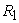and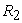. If they are compressed to each other by a normal force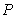, than the radius of contact surface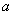is expressed by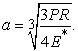(1)

If the reduced elastic modulus is equal to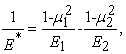(2)

where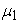and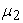are the Poisson ratios,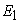and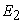are the elastic moduli and the relative radius is defined as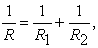(3)

than the value of contact pressure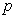as a function of radius can be assumed as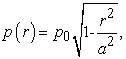(4)

whereby the maximal value of Hertzian pressure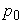is equal to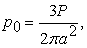(5)

and the contact surface area is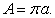(6)
2.2. Contact of Cylindrical-Shaped Bodies

The relations for contact calculation of two cylinders are similar to those, used by spheres. The only difference is that the contact surface has a rectangular shape with a width of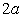. For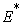and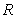defined equally to the previous case, the half width of contact surface can be expressed by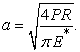(7)

The contact pressure as a function of widthis given by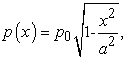(8)

whereby the maximal value of Hertzian pressure is equal to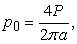(9)

and the contact surface area for a cylinder with a length of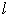is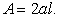(10)

Hertzian model is characterized as sufficiently suitable for the description of contact area by the absence of adhesion. A lot of experiments as well as theoretical computations prove that the influence of adhesion acting between the body surfaces cannot be neglected. In fact, the contact area is due to the existence of these forces much higher as considered by Hertz. As he noted in his work, the radius of the contact surface can be expressed by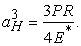(11)

For simplification let us consider a quantity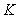, given by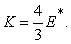(12)

Then the radius of contact surface can be expressed in simplified form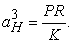(13)

The value of adhesive force, acting between two rigid round bodies is equal to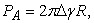(14)

where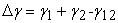signifies the adhesion work, whereby the parameters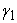and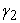are the surface energies of the materials, which are the bodies made from and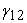is the energy between the surfaces. Eq. (14) is valid for rigid bodies and thus the radius of contact surface should be equal to zero. In fact the bodies will deform during contact. Derjaguin, Muller and Toporov created a model for deformable bodies. After substituting eq. (14) into eq. (11) the radius of contact surface is given by a relation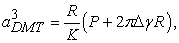(15)

which is known as DMT model.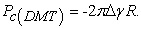(16)

In this case the radius of contact surface is equal to zero, what means that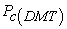is a force needed for separation of two spheres. If this force is equal to zero, the contact surface is given by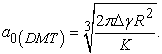(17)

The other approach, which considers adhesive forces by contact, was introduced by Johnson, Kendall and Roberts, known as JKR model. It concerns with a contact between a rigid round body and a deformable half-space. According to this theory the radius of contact surface is expressed as a function of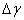by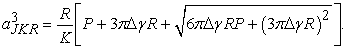(18)

The body surfaces will be separated applying the force, which value is given by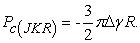(19)

The model considers, that if this force equals to zero, the contact surface will be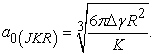(20)

From eq. (19) it is obvious that the force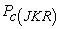is not a function of elastic modulus, but i tis dependent on adhesion work and reduced radius. In this case this equation should be applied also for rigid bodies. However, it contradicts the eq. (16). Muller finally stated that both models are only the marginal extremes of non-dimensional parameter given by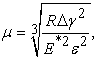(21)

where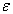is the equilibrium distance in the Lennard-Jones potential,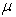is the elastic deformation ratio resulting from adhesion and effective range of surface forces. Afterwards Maugis introduced the other non-dimensional quantity – transition parameter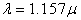.

Maugis model can be applied for each value of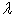, thus it is more accurate JKR model is suitable for solving contact of deformable bodies with high radius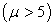and DMT model for rigid bodies with small radius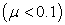.

### 4. Experimental Contact Mechanics Using Digital Image Correlation Method

The measurement was performed on a specimen, which consisted of two independent bodies, which shapes and dimensions are depicted in Figure 2.Download asVeiw figureFigures index
Figure 2. Shapes and dimensions of two bodies creating the specimen

Part of the specimen, labeled as 1, should present a model of cantilever beam attached to a wall 2. The specimen was created from the PSM-1 material, used in transmission photoelasticity method, via water jet technology, in order to eliminate a possibility of the residual stresses appearing on the model boundaries. The mechanical properties of mentioned material are: E = 2500 MPa, η = 0,38, Rp0,2 = 80 MPa, ρ = 1280 kg.m-3.

The specimen was analyzed in laboratory conditions, whereby the loading was due to a system with lever mechanism (see Figure 3). The part 1 of the specimen was loaded by a force, changing by the increment of 10 N from 0 N up to 70 N, acting on a small surface nearly on its free end.Download asVeiw figureFigures index
Figure 3. Specimen located in the loading system with a lever mechanism

The measurement was realized using digital image correlation method. A low-speed digital image correlation system Q-400 Dantec Dynamics was used to capture a reference state of the specimen without loading and compare (correlate) it with the remaining loading states. This system consists of two CCD cameras with the resolution of 5 Mpx and maximal sampling frequency of 2 fps, that allows to use it in 3D static strain analysis. As the aim of the measurement was to evaluate a contact mechanics problem, the cameras were located in the distance of ca. 515 mm of the specimen and focused on the area depicted in Figure 4.Download asVeiw figureFigures index
Figure 4. The view on the investigated specimen surface captured by one of the correlation system CCD cameras

The calibration of the cameras was realized using the checkered calibration target with 9x9 black and white fields of the size of 11 mm. The facet size used for evaluation was set to 10x10 px. By such adjusted correlation parameters, the system evaluates 85627 facets on the investigated object surface. The acquired data in a form of displacements and strains were imported into application Q-STRESS v.1.0 in order to calculate and visualize the stress fields. Von Mises stress field obtained in mentioned application by maximal loading force of 70 N is depicted in Figure 5. On the evaluate surface of the model part 1, ten locations were chosen, in which the values of Guest stress were compared to the values of Guest stress in corresponding points using finite element analysis.Download asVeiw figureFigures index
Figure 5. Guest stress field acquired by Q-STRESS v.1.0 with the virtual gages on the model surface

### 5. Numerical Contact Mechanics Using Finite Element Analysis

For verification of the acquired results a static analysis in Ansys Workbench was realized. By such analysis it is necessary to define correctly the contacts between both parts of the numerical model. Three faces of both model parts, which touch themselves, were chosen to define on them frictionless – solid to solid type of contact (see Figure 6). According to Chen and Liu  frictionless contact model allows free sliding, assuming a zero coefficient of friction. Gap can form in between regions in contact.

The elements’ size was adjusted to the size of the model and set to 1.5 mm. All the elements were hexahedrons with program controlled element midside nodes. Thus the model created in Ansys consisted of 35160 elements with 167198 nodes.

To ensure the boundary conditions corresponding to the experimental investigation displacements on three faces of the part 2 were constrained. On its right side (in Figure 6 denoted as A) the displacements in direction x and z were constrained. Moreover, on its upper as well as bottom side the displacements in direction x and y were constrained (in Figure 6 denoted as B). The force of 70 N acted on a small surface created nearly the left edge of the part 1 (in Figure 6 denoted as C).Download asVeiw figureFigures index
Figure 6. The boundary conditions created on the numerical model

The Guest stress field on the area of interest acquired in Ansys Workbench is depicted in Figure 7. The field concerns also the probes, which should be located approximately in the same locations as in the experimental model.Download asVeiw figureFigures index
Figure 7. Guest stress field acquired by Ansys Workbench with the virtual gages on the model surface

### 6. Discussion

As can be seen from Figure 5 and Figure 7 the distributions of Guest stress acquired by experimental and numerical modeling correspond relatively good together. The difference can be seen in the locations, where the contacts between both parts of the model occur. The reason is that Ansys Workbench considers with a point contact, however, in real, there is a contact on a small surface (see the red colored surfaces in the results of experimental investigation).

A quantitative comparison of the acquired results was realized in the chosen virtual gages, in which the values of Guest stress, given in Table 1. were acquired. The courses of the mentioned Guest stress values are depicted in Figure 8.

#### Table 1. Guest stress values obtained in virtual gagesDownload asPowerPoint Slide

Veiw figure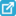View current table in a new windowDownload asVeiw figureFigures index
Figure 8. Courses of the Guest stress acquired in virtual gages

### 7. Summary

Digital image correlation is a modern optical full-field method applicable in wide range of mechanical problems. The contribution describes methodology for experimental investigation of the Guest stress in a simple contact mechanics problem. For the realization of the experiment a low-speed correlation system Q-400 Dantec Dynamics with additional application Q-STRESS v.1.0, developed at the authors’ department, were used.

Mentioned experimental stress analysis confirmed a good correspondence with the results acquired by numerical modeling using Ansys Workbench, when the maximal difference between determined Guest stress values was approximately 10 %.

### Acknowledgements

The paper is the result of the project implementation VEGA 1/0731/16.

### References

  Johnson K. L., Kendall K. and Roberts A. D., “Surface energy and the contact of elastic solids,” Proceedings of the Royal Society A: Mathematical, Physical and Engineering Sciences, 324(1558), 1971.In article View Article  Derjaguin B. V., Muller V. M. and Toporov Yu. P., Effect of contact deformations on the adhesion of particles, Progress in Surface Science, 45(1-4), p. 131-143, 1994.In article View Article  G. W. Stachowiak and A. W. Batchelor, Engineering Tribology (Fourth Edition), Butterworth-Heinemann, 2013.In article  R. Gohar and H. Rahnejat, Fundamentals of Tribology, Imperial College Press, 2008.In article View Article  P. Menezes, S. P. Ingole, M. Nosonovsky, S. V. Kailas, M. R. Lovell, Tribology for Scientists and Engineers: From Basics to Advanced Concepts, Springer, 2013.In article View Article  B. Bhushan, Modern Tribology Handbook (Two Volume Set), 3rd Chapter, CRC Press, 2000In article View Article  X. Chen and Y. Liu, Finite Element Modeling and Simulation with ANSYS Workbench, CRC Press, 2014.In article